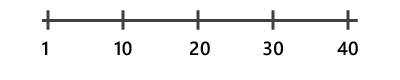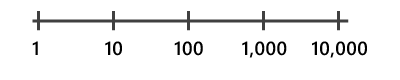# Scatter plot

Scatter plots visualize the relationship between two numeric variables, where one variable is displayed on the x-axis, and the other variable is displayed on the y-axis. For each record, a point is plotted where the two variables intersect in the chart. When the resulting points form a nonrandom structure, a relationship exists between the two variables.

## Variables

Scatter plots are made up of two Numbers, one for the x-axis and one for the y-axis. Additionally, a third numeric variable can be specified to proportionally size each point in the plot.

## Statistics

A regression equation is calculated and the associated trend line and R² are plotted on scatter plots. The trend line models the linear relationship between x and y, and the R² quantifies how well the data fits the model. This is only relevant for linear relationships. To turn off the trend line, uncheck the Show linear trend checkbox in the Chart Properties pane, or toggle visibility by clicking the item in the legend. To change the color of the trend line, click the trend line color swatch in the Chart Properties and choose a new color.

## Correlation

When small x values correspond to small y values, and large x values correspond to large y values (line sloping up), this indicates a positive correlation. When small x values correspond to large y values, and large x values correspond to small y values (line sloping down), this indicates a negative correlation.

##### Note:

It is important to note that x being correlated to y does not imply that x causes y.

## Symbol

### Size

Scatter plot points can be uniform in size, or sized proportionally by a numeric attribute. Sizing scatter plot points proportionally based on a third numeric variable adds an additional dimension to the visualisation, creating a bubble plot.

### Color

Scatter plot points can be visualized using a single color, or with the colors specified in the layer's symbology. By default, scatter plots use layer colors and inherit their outline and fill colors from the source layer symbology. By symbolizing a layer with a different attribute than either of the scatter plot variables, an additional dimension can be shown on the scatter plot visualization.

## Axes

### Axis bounds

Default minimum and maximum axis bounds are set based on the range of data values represented on the axis. These values can be customized by typing in a new desired axis bound value. Clicking the reset icon will revert the axis bound back to the default value.

### Log axis

By default, scatter plot axes are displayed on a linear scale. One or both axes can be displayed on a logarithmic scale by checking the Log axis checkbox in the Axes section of the Chart Properties pane.

Logarithmic scales are useful when visualizing data with large positive skew, where the bulk of data points have a small value, with a few data points with very large values. Changing the scale of the axis does not change the value of the data, just the way it is displayed.

Linear scales are based on addition, and logarithmic scales are based on multiplication.

On a linear scale, each increment on the axis represents the same distance in value. For example, in the axis diagram below, each increment on the axis increases by adding 10.On a logarithmic scale, increments increase by magnitudes. In the axis diagram below, each increment on the axis increases by multiplying by 10.##### Note:

Logarithmic scales cannot display negative values or zero. If you chose to log the axis of a variable with negative values or zero, those values will not appear on the chart.

### Number format

You can format the way an axis will display numeric values by specifying a number format category or by defining a custom format string. For example, \$#,### can be used as a custom format string to display currency values.

## Appearance

### Titles and description

Charts and axes are given default titles based on the variable names and chart type. These can be edited on the General tab in the Chart Properties pane. You can also provide a chart Description, which is a block of text that appears at the bottom of the chart window.

## Guides

Guide lines or ranges can be added to charts as a reference or way to highlight significant values. To add a new guide, navigate to the Guides tab in the Chart Properties pane, choose if you would like to draw a vertical or horizontal guide, and click Add guide. To draw a line, enter a Value where you would like the line to draw. To create a range, enter a to value. You can optionally add text to your guide by specifying a Label.

## Example

Create a scatter plot to visualize the relationship between diabetes and hypertension among Medicare beneficiaries. Select features in the chart to see where they fall on the map.

• X-Axis— Diabetes rate
• Y-Axis— Hypertension rate